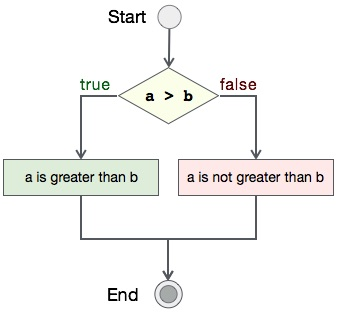# Progra to read file in C

## Algorithm

Let's first see what should be the step-by-step procedure to compare two integers−

```START

Step 1 → Take two integer variables, say A & B

Step 2 → Assign values to variables

Step 3 → Compare variables if A is greater than B

Step 4 → If true print A is greater than B

Step 5 → If false print A is not greater than B

STOP
```

## Flow Diagram

We can draw a flow diagram for this program as given below −## Pseudocode

Let's now see the pseudocode of this algorithm −

```procedure compare(A, B)

IF A is greater than B
DISPLAY "A is greater than B"
ELSE
DISPLAY "A is not greater than B"
END IF

end procedure
```

## Implementation

Assuming we have file with name file_append.txt with this content −

```You have opened a file using C programming language, in read-only mode.
```

Now, we shall see the actual implementation of the program −

```#include <stdio.h>

int main()
{
FILE *fp;
char ch;

/*  open for writing */
fp = fopen(filename, "r");

if (fp == NULL)
{
printf("%s does not exists \n", filename);
return;
}
else
{
printf("%s: opened in read mode.\n\n", filename);
}

while ((ch = fgetc(fp) )!= EOF)
{
printf ("%c", ch);
}

if (!fclose(fp))
printf("\n%s: closed.\n", filename);

return 0;
}
```

## Output

Output of this program should be −

```file_read.txt: opened in read mode.

You have opened a file using C programming language, in read-only mode.